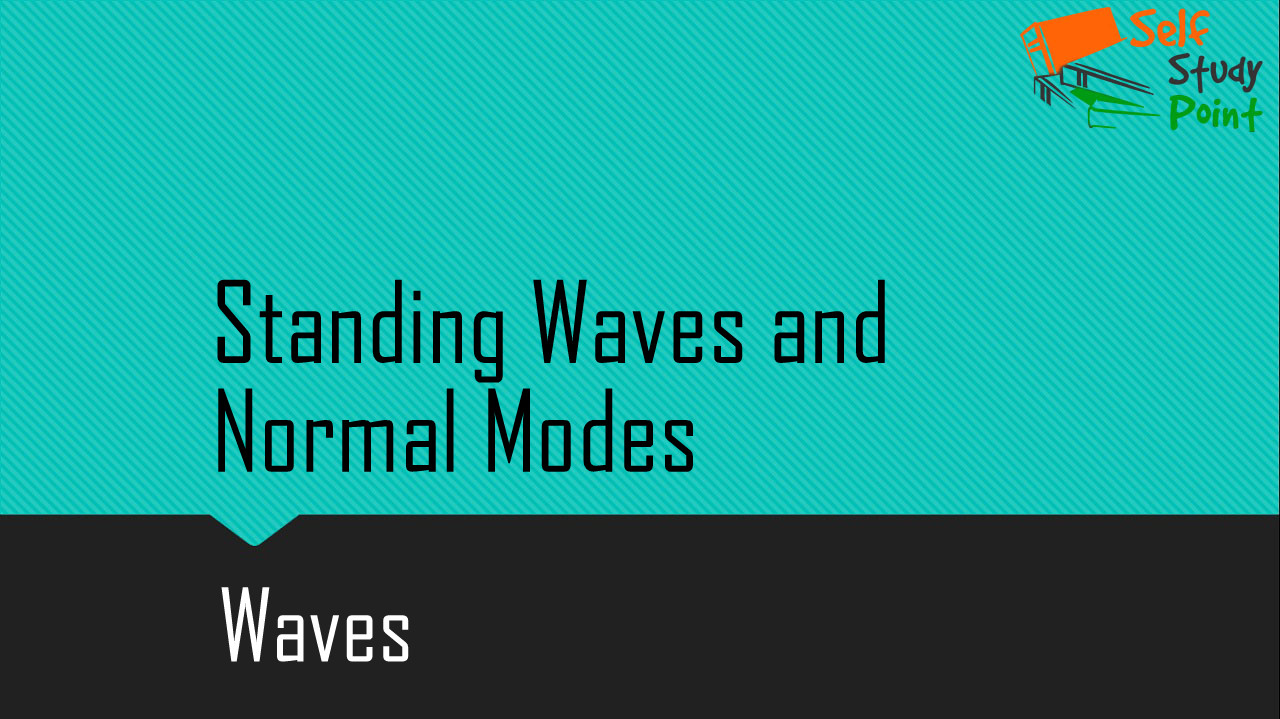The interference of two identical waves moving in opposite directions produces standing waves. For a string with fixed ends, the standing wave is given by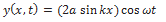Standing waves are characterized by fixed locations of zero displacement called nodes and fixed locations of maximum displacements called antinodes. The separation between two consecutive nodes or anti-nodes is λ/2.

For a stretched string of length L, fixed at both ends, the two ends of the string have to be nodes. If one of the ends is chosen as position x = 0, then the other end is x = L. In order that this end is a node; the length L must satisfy the conditionThis condition shows that standing waves on a string of length L have restricted wavelength given by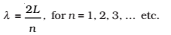The frequencies corresponding to these wavelengths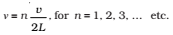where v is the speed of traveling waves on the string.

The set of frequencies given by the above relation are called the normal modes of oscillation of the system. The oscillation mode with the lowest frequencyis called the fundamental mode or the first harmonic.

The second harmonic is the oscillation mode with n = 2 and so on.

Put in above equation,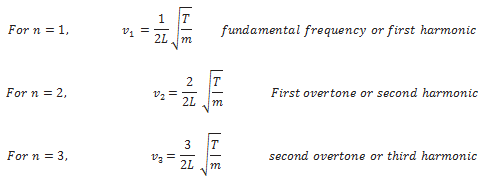The set of frequencies represented by the above relation are the normal modes of oscillation of such a system. The lowest frequency given by v/4L is the fundamental mode or the first harmonic.

Click on a star to rate it!

Average rating 1 / 5. Vote count: 1

No votes so far! Be the first to rate this post.

As you found this post useful...